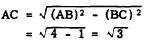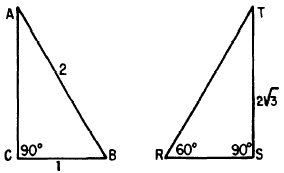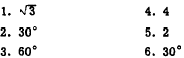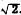Custom SearchRIGHT TRIANGLES WITH SPECIAL ANGLES AND SIDE RATIOS Three types of right triangles are especially significant because of ‘their frequent occurrence. These are the 30°-60°-90° triangle, the 45°-90° triangle, and the 3-4-5 triangle. THE 30°-60°-90° TRIANGLE The 30°-60°-90° triangle is so named because these are the sizes of its three angles. The sides of this triangle are in the ratio of 1 toto 2, as shown in figure 19- 10.Figure 19-lo.-30°-60°-90" triangle. The sine ratio for the 30° angle in figure 19-10 establishes the proportionate values of the sides. For example, we know that the sine of 30° is 1/2; therefore side AB must be twice as long as BC. If side BC is 1 unit long, then side AB is 2 units long and, by the rule of Pythagoras, AC is found as follows:Regardless of the size of the unit, a 30°- 60°-90° triangle has a hypotenuse which is 2 times as long as the shortest side. The shortest side is opposite the 30° angle. The side opposite the 60° angle is fl times as long as the shortest side. For example, suppose that the hypotenuse of a 30°-60°-90° triangle is 30 units long; then the shortest side is 15 units long, and the length of the side opposite the 60° angle is 15units. Practice problems. Without reference to tables or to the rule of Pythagoras, find the following lengths and angles in figure 19-11: 1. Length of AC. 2. Size of angle A. 3. Size of angle B. 4. Length of RT. 5. Length of RS. 6. Size of angle T.Figure 19-11.-Finding parts of 30°-60°-90° triangles. Answers:THE 45°-90° TRIANGLE Figure 19-12 illustrates a triangle in which two angles measure 45° and the third angleFigure 19-12.-A 45°-90° triangle. measures 90°. Since angles A and B are equal, the sides opposite them are also equal. Therefore, AC equals CB. Suppose that CB is 1 unit long; then AC is also 1 unit long, and the length of AB is calculated as follows:Regardless of the size of the triangle, if it has two 45° angles and one 90° angle, its sides are in the ratio 1 to 1 to. For example, if sides AC and CB are 3 units long, AB is 3units long. Practice problems. Without reference to tables or to the rule of Pythagoras, find the following lengths and angles. in figure 19-13: 1. AB  2. BC  3. Angle B Answers: 1. 22. 2  3. 45°